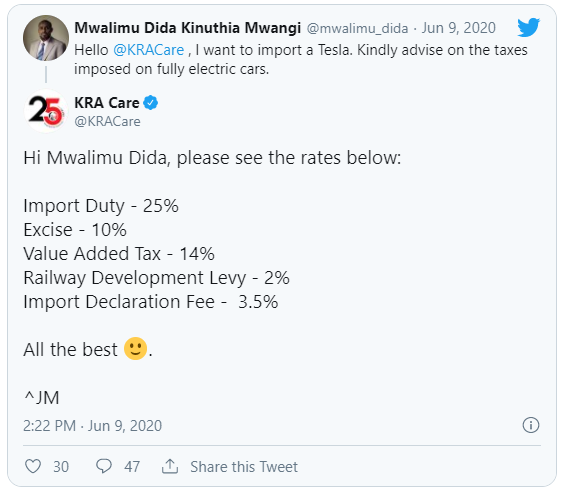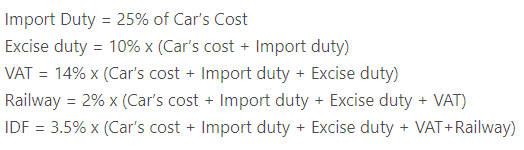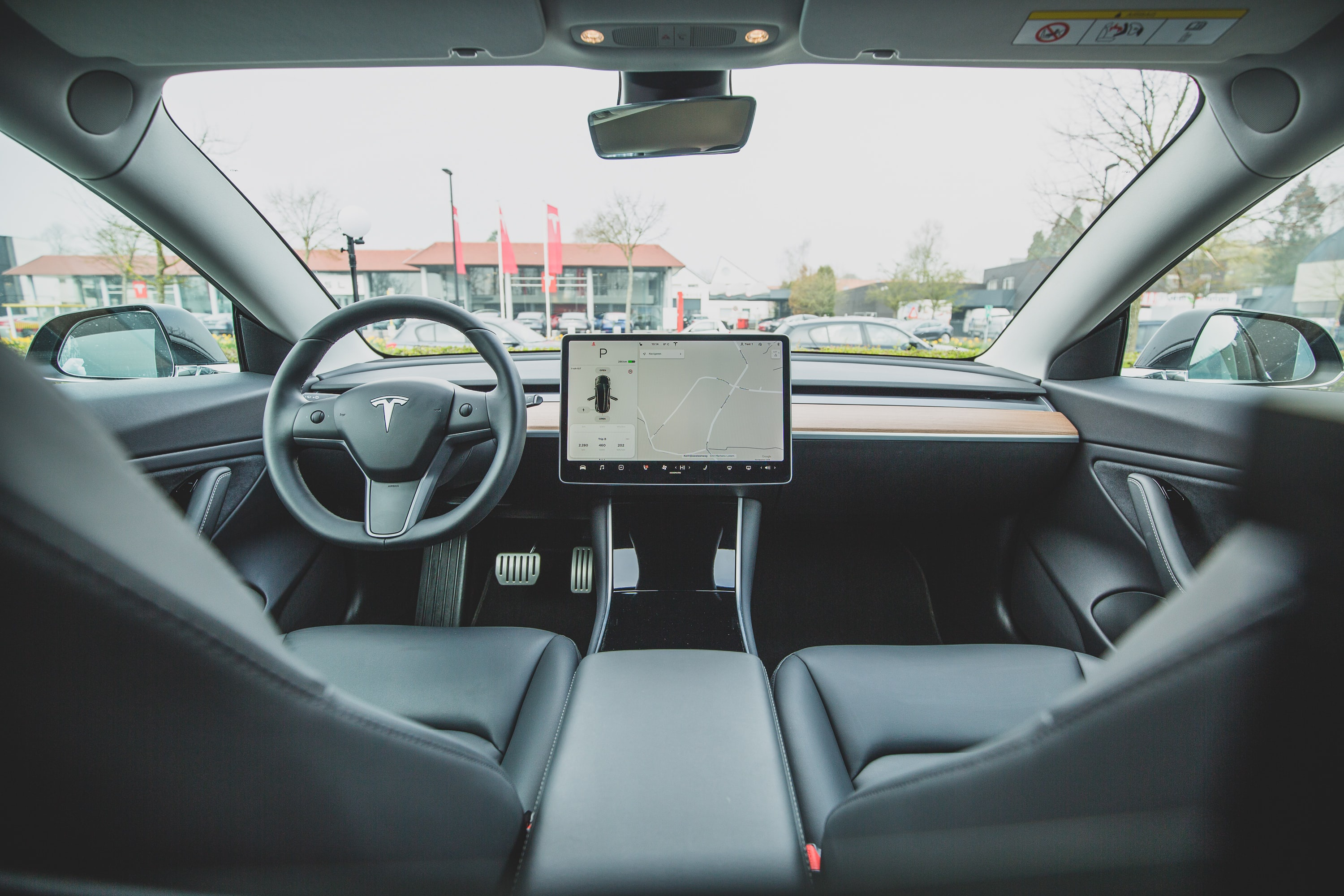# How Much Would a Tesla Model 3 Cost in Kenya?Image by Robin Van Geneen Via Unsplash

In February this year, the new Tesla Model X in Kenya created a lot of Buzz. Kenyans had a lot of mixed reactions.

How would the car be serviced? Why did the Patel Family settle for the Tesla rather than another brand like Toyota V8 or a Range Rover?

Viju Patel's answer was that he wanted the best electric car in the market and Tesla managed to be in his list.

The first-mover advantage into the electric car space has given Tesla huge market attention thereby allowing the company to grow at a rapid pace.

The model X bought by Viju Patel is said to have cost about Kshs 15 Million. The question that most people still have is, how much would a Tesla Model 3 cost if imported to Kenya?

In 2020, KRA gave the tax rates for importing an electric car.In simple terms, the above rates would be calculated as shown below.Going with the cheapest Tesla Model 3 which is the Standard Range Plus, the normal cost would be 4 million without taxes.Image by Bram Van Oost Via Unsplash

Including the taxes indicated by KRA above, the price of a Tesla Model 3 would go up as follows;

Import Duty = (25% of 4,000,000) = 1,000,000

Exercise Duty = 10% * (4,000,000+1,000,000) = 500,000

VAT = 14% * (4,000,000 + 1,000,000 + 500,000) = 770,000

Railway = 2% * (4,000,000 + 1,000,000 + 500,000 + 770,000) = 125,400

IDF = 3.5% * (4,000,000 + 1,000,000 + 500,000 + 770,000 + 125,400) = 223,839

The total cost would be (4,000,000 + 1,000,000 + 500,000 + 770,000 + 125,400 + 223,839) = 6,619,239

From the calculation, the Tesla Model 3 would therefore cost around Kshs 6.6 Million or close to Kshs 7 Million.

Being among the best electric cars in the market, the price for purchasing Tesla Model 3 would be termed as average.

Would you pay the price for a new Tesla Model 3? or would you go for another car brand? Leave a comment below and let's keep the discussion going on.